The COUNTREG Procedure

Overview: COUNTREG Procedure

The COUNTREG (count regression) procedure analyzes regression models in which the dependent variable takes nonnegative integer or count values. The dependent variable is usually an event count, which refers to the number of times an event occurs. For example, an event count might represent the number of ship accidents per year for a given fleet. In count regression, the conditional mean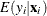of the dependent variableis assumed to be a function of a vector of covariates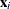.

The Poisson (log-linear) regression model is the most basic model that explicitly takes into account the nonnegative integer-valued aspect of the outcome. With this model, the probability of an event count is determined by a Poisson distribution, where the conditional mean of the distribution is a function of a vector of covariates. However, the basic Poisson regression model is limited because it forces the conditional mean of the outcome to equal the conditional variance. This assumption is often violated in real-life data. Negative binomial regression is an extension of Poisson regression in which the conditional variance can exceed the conditional mean. Also, a common characteristic of count data is that the number of zeros in the sample exceeds the number of zeros that are predicted by either the Poisson or negative binomial model. Zero-inflated Poisson (ZIP) and zero-inflated negative binomial (ZINB) models explicitly model the production of zero counts to account for excess zeros and also enable the conditional variance of the outcome to differ from the conditional mean.

In zero-inflated models, additional zeros occur with probability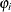, which is determined by a separate model,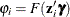, where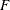is the normal or logistic distribution function that results in a probit or logistic model and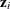is a set of covariates.

PROC COUNTREG supports the following models for count data:

• Poisson regression

• Conway-Maxwell-Poisson regression

• negative binomial regression with quadratic (NEGBIN2) and linear (NEGBIN1) variance functions (Cameron and Trivedi, 1986)

• zero-inflated Poisson (ZIP) model (Lambert, 1992)

• zero-inflated Conway-Maxwell-Poisson (ZICMP) model

• zero-inflated negative binomial (ZINB) model

• fixed-effects and random-effects Poisson models for panel data

• fixed-effects and random-effects negative binomial models for panel data

The count data models have been used extensively in economics, political science, and sociology. For example, Hausman, Hall, and Griliches (1984) examine the effects of research and development expenditures on the number of patents obtained by U.S. companies. Cameron and Trivedi (1986) study factors that affect the number of doctor visits that a group made during a two-week period. Greene (1994) studies the number of derogatory reports to a credit reporting agency for a group of credit card applicants. As a final example, Long (1997) analyzes the number of publications by Ph.D. candidates in science in the final three years of their doctoral studies.

The COUNTREG procedure can use the maximum likelihood method and the Bayesian method. Initial starting values for the nonlinear optimizations are typically calculated by OLS. When a model that contains a dependent count variable is estimated using linear ordinary least squares (OLS) regression, the count nature of the dependent variable is ignored. This can lead to negative predicted counts and to parameter estimates that have undesirable properties in terms of statistical efficiency, consistency, and unbiasedness unless the mean of the counts is high, in which case the Gaussian approximation and linear regression might be satisfactory.